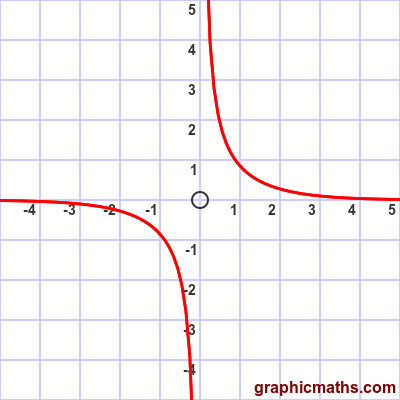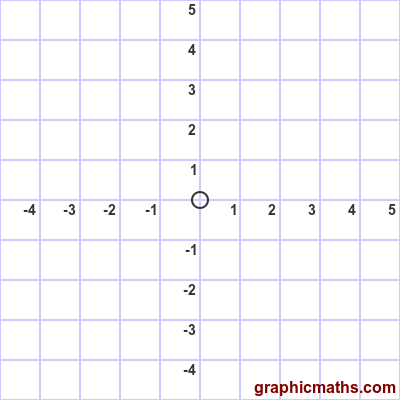# cosech function

By Martin McBride, 2021-02-04
Tags: cosech sinh inverse hyperbolic function
Categories: hyperbolic functions pure mathematics

The cosech function is a hyperbolic function. It is also known as the hyperbolic cosecant function.

Here is a video that explains the cosech, sech, and coth functions:

## Equation and graph

The cosech function is the reciprocal of the sinh function, and is only defined for non-zero values:

$$\operatorname{cosech}{x} = \frac{1}{sinh{x}}$$

(This is analogous with trig functions, where cosec is the reciprocal of sin.)

Substituting the formula for sinh gives:

$$\operatorname{cosech}{x} = \frac{2}{e^{x}-e^{-x}}$$

Here is a graph of the function:## Sketching cosech

We can sketch the cosech curve based on the sinh curve:The sinh curve tends to -∞ for large negative $x$, it passes through the origin at $x = 0$, and it tends to +∞ for large positive $x$.

The cosech curve is the reciprocal of sinh. It tends to zero for large negative $x$, and tends to -∞ as $x$ approaches zero from the negative direction. It also tends to zero for large positive $x$, and but tends to +∞ as $x$ approaches zero from the positive direction.

## Other forms of the equation

If we take the two alternative forms of the sinh function, and invert them, we get two alternative forms of the cosech function:

$$\operatorname{cosech}{x} = \frac{2e^{x}}{e^{2x}-1}$$

$$\operatorname{cosech}{x} = \frac{2e^{-x}}{1-e^{-2x}}$$